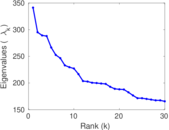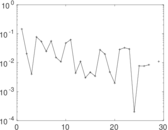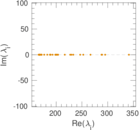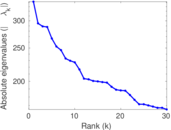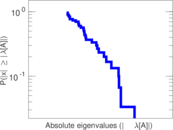This network consists of the wikilinks of the Wikipedia in the Swedish language (sv). Nodes are Wikipedia articles, and directed edges are wikilinks, i.e., hyperlinks within one wiki. In the wiki source, these are indicated with [[double brackets]]. Only pages in the article namespace are included.

 Code `Wsv` Internal name `wikipedia_link_sv` Name Wikipedia links (sv) Data source http://dumps.wikimedia.org/ AvailabilityDataset is available for download Consistency checkDataset passed all tests Category Hyperlink network Node meaning Article Edge meaning Wikilink Network format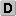Unipartite, directed Edge typeUnweighted, no multiple edges ReciprocalContains reciprocal edges Directed cyclesContains directed cycles Loops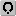Contains loops

## Statistics

 Size n = 6,100,692 Volume m = 106,749,786 Loop count l = 1,476 Wedge count s = 31,734,209,367,649 Claw count z = 1.408 28 × 1019 Cross count x = 5.600 29 × 1024 Triangle count t = 682,865,621 Maximum degree dmax = 2,732,819 Maximum outdegree d+max = 3,981 Maximum indegree d−max = 2,732,806 Average degree d = 34.996 0 Fill p = 2.868 20 × 10−6 Size of LCC N = 6,100,574 Diameter δ = 10 50-Percentile effective diameter δ0.5 = 2.668 99 90-Percentile effective diameter δ0.9 = 3.928 84 Median distance δM = 3 Mean distance δm = 3.229 81 Balanced inequality ratio P = 0.238 778 Outdegree balanced inequality ratio P+ = 0.311 963 Indegree balanced inequality ratio P− = 0.074 159 4 Clustering coefficient c = 6.455 48 × 10−5 Directed clustering coefficient c± = 0.171 679 Operator 2-norm ν = 4,907.13 Reciprocity y = 0.125 868 Non-bipartivity bA = 0.003 068 33 Normalized non-bipartivity bN = 0.028 943 5

## Plots

### Degree distribution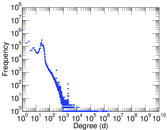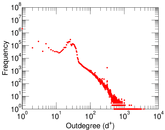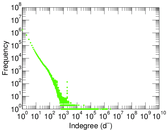### Cumulative degree distribution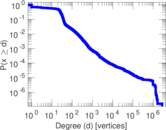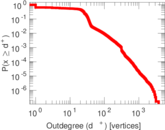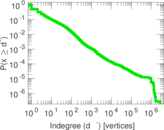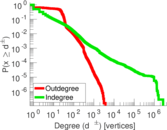### Hop distribution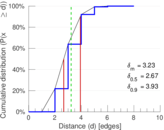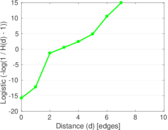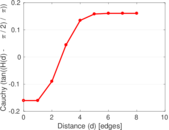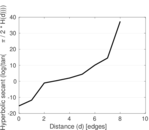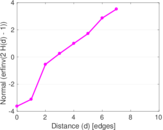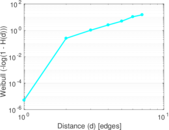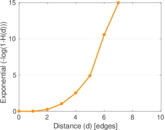### In/outdegree scatter plot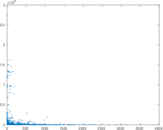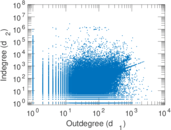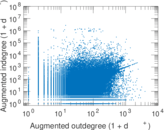### Matrix decompositions plots